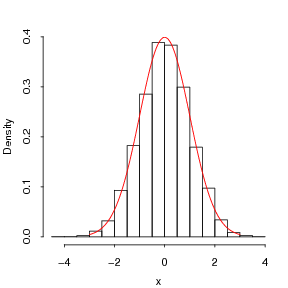Want to share your content on R-bloggers? click here if you have a blog, or here if you don't.

# Acceptance-rejection methods

This post is based on chapter 1.4 of Advanced Markov Chain Monte Carlo.

Another method of generating random variates from distributions is to use acceptance-rejection methods. Basically to generate a random number from$f(x)$, we generate a RN from an envelope distribution$g(x)$, where$sup f(x)/g(x) le M le infty$.  The acceptance-rejection algorithm is as follows:

Repeat until we generate a value from step 2:

1. Generate$x$ from$g(x)$ and$U$ from$Unif(0, 1)$

2. If$U le frac{f(x)}{M g(x)}$, return$x$ (as a random deviate from$f(x)$).

## Example: the standard normal distribution

This example illustrates how we generate$N(0, 1)$ RNs using the logistic distribution as an envelope distribution. First, note that$displaystyle f(x) = frac{1}{2pi} e^{-x^2/2} quad mbox{and} quad g(x) = frac{e^{-x/s}}{s(1+ e^{-x/s})^2}$

On setting$s=0.648$, we get$M = 1.081$. This method is fairly efficient and has an acceptance rate of$displaystyle r = frac{1}{M}frac{int f(x) dx}{int g(x) dx} = frac{1}{M} = 0.925$

since both$f$ and$g$ are normalised densities.

### R code

This example is straightforward to code:

```myrnorm = function(M){
while(1){
u = runif(1); x = rlogis(1, scale = 0.648)
if(u < dnorm(x)/M/dlogis(x, scale = 0.648))
return(x)
}
}
```

To check the results, we could call `myrnorm` a few thousand times:

```hist(replicate(10000, myrnorm(1.1)), freq=FALSE)
lines(seq(-3, 3, 0.01), dnorm(seq(-3, 3, 0.01)), col=2)
```## Example: the standard normal distribution with a squeeze

Suppose the density$f(x)$ is expensive to evaluate. In this scenario we can employ an easy to compute function$s(x)$, where$0 le s(x) le g(x)$ .$s(x)$ is called a squeeze function. In this example, we’ll use a simple rectangular function, where$s(x) = 0.22$ for$x=-1, ldots, 1$. This is shown in the following figure:

Repeat until we generate a value from step 2:

1. Generate$x$ from$g(x)$ and$U$ from$Unif(0, 1)$

2. If$U le frac{s(x)}{M g(x)}$ or$U le frac{f(x)}{M g(x)}$, return$x$ (as a random deviate from$f(x)$).

Hence, when$U le frac{s(x)}{M g(x)}$ we don’t have to compute$f(x)$. Obviously, in this example$f(x)$ isn’t that difficult to compute.